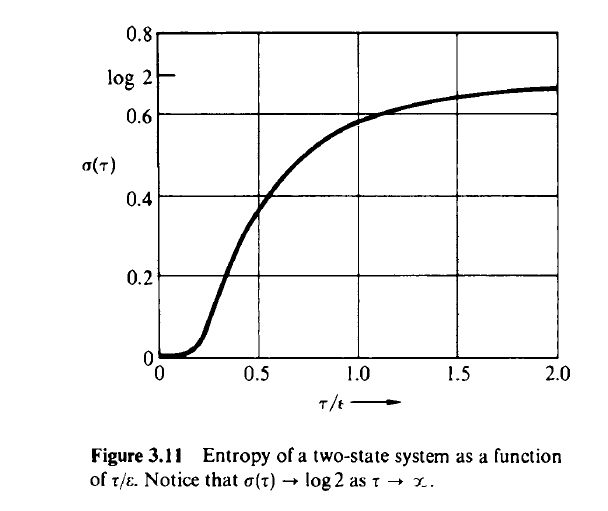# Expressions for energy & entropy from free energy (discrete distribution)

Homework Statement:
(a) Find an expression for the free energy as a function of ##\tau## (temperature) of a system with two states, one at energy 0 and one at energy ##\epsilon##. (b) From the free energy, find expressions for energy and entropy of the system. The entropy is plotted in Figure 3.11 (see below).
Relevant Equations:
$$Z = \sum_i e^{ frac {-E_i} {k_BT} }$$
$$F = -k_B T \ln{Z}$$
$$F = U - TS$$So just by by using the definition of the partition function...
$$Z = \sum_i e^{ \frac {-E_i} {k_BT} } = e^{ \frac {-0} {k_BT} } + e^{ \frac {-\epsilon} {k_BT} } = 1 + e^{ \frac {-\epsilon} {k_BT} }$$

And then, a result we obtained in class by using the Boltzmann H factor to solve for ##S## and finding an expression for ## U - TS## gave us ##F = -k_B T \ln{Z} ##. So applying that gives part (a)...

$$F = -k_B T \ln{ 1 + e^{ \frac {-\epsilon} {k_BT} } }$$

Now for (b), I'm confused. I'm not sure if I have an equation to get S and U out of just this free energy expression. All I have is the free energy definition, ## F = U - TS ##, and if I try to solve using that and my above expression for free energy, I'm going to get something like ## U = U ## or ## S = S##. So I'm not sure which equation/principle to use. I have thought about using differential with constant volume but I don't think that would help and the question seems pretty explicit about using the free energy expression from (a) to obtain both U and S.

TSny
Homework Helper
Gold Member
Think of ##U## as ##\langle E \rangle##. For the two-state system, how would you calculate ##\langle E \rangle##? Hint: What is the probability that the system has energy 0? What is the probability the system has energy ##\epsilon##?

Think of ##U## as ##\langle E \rangle##. For the two-state system, how would you calculate ##\langle E \rangle##? Hint: What is the probability that the system has energy 0? What is the probability the system has energy ##\epsilon##?

Oh ok. So ...
$$\langle E \rangle = \sum_{E = 0, E = \epsilon} E*P(E) = \frac {e^{\frac {0} {k_BT}}} {Z}*0 + \frac {e^{\frac {-\epsilon} {k_BT}}} {Z} *\epsilon$$

$$\langle E \rangle = \frac {e^{\frac {-\epsilon} {k_BT} } } {1 + {e^{\frac {-\epsilon} {k_BT} } } *\epsilon$$

Is this the expression for "the energy of the system" that (b) is asking me for? So then I plug this into ## F = U - TS## to solve for entropy?

TSny
Homework Helper
Gold Member
Yes

•baseballfan_ny
That's interesting, I would have that by "energy of the system," they mean some sort of general expression, like energy as a function of the state. But it actually refers to an ensemble average of energy. Is there a reason for that? My guess is it has something to do with the system being discrete.

TSny
Homework Helper
Gold Member
That's interesting, I would have that by "energy of the system," they mean some sort of general expression, like energy as a function of the state. But it actually refers to an ensemble average of energy. Is there a reason for that? My guess is it has something to do with the system being discrete.
I'm not sure I understand what you are asking here. Taking the thermodynamic ##U## to correspond to the statistical mechanics ##\langle E \rangle## is standard, I believe.

Another approach to the problem is to first find ##S## using the thermodynamic relation ##S = -\frac {\partial F}{\partial T}##. Then you can find ##U## from ##F = U - TS##.

Or, maybe you have seen the relation ##U= - \large \frac {\partial \ln Z}{\partial \beta}##, where ##\beta = \large \frac 1 {kT}##. This can be used to find ##U##.

•baseballfan_ny
Taking the thermodynamic U to correspond to the statistical mechanics ⟨E⟩ is standard, I believe.
That's exactly what I was asking :) Now that I think about, I think our instructor had mentioned it once briefly during class. Also got the same answer from trying the other two methods. Thanks for the help.

•TSny